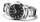# Chocolate

Children break chocolate first to third and then every part of another half. What kind got each child? Draw a picture. What part would have received if each piece have halved?

Result

x =  0.167
y =  0.084

#### Solution:Leave us a comment of example and its solution (i.e. if it is still somewhat unclear...):

Showing 0 comments:Be the first to comment!#### To solve this example are needed these knowledge from mathematics:

Need help calculate sum, simplify or multiply fractions? Try our fraction calculator.

## Next similar examples:

1. In fractionsAn ant climbs 2/5 of the pole on the first hour and climbs 1/4 of the pole on the next hour. What part of the pole does the ant climb in two hours?
2. CakesOn the bowl were a few cakes. Jane ate one-third of them, Dana ate a quarter of those cakes that remained. a) What part (of the original number of cakes) Dana ate? b) At least how many cakes could be (initially) on thebowl?
3. Math classificationIn 3A class are 27 students. One-third got a B in math and the rest got A. How many students received a B in math?
4. ZdeněkZdeněk picked up 15 l of water from a 100-liter full-water barrel. Write a fraction of what part of Zdeněk's water he picked.
5. MinutesWrite as fraction in basic form which part of the week is 980 minutes.
6. Fraction and a decimalWrite as a fraction and a decimal. One and two plus three and five hundredths
7. Cake 71/3 of a cake shared with 4 people. What share of the whole cake has each people?
8. The resultHow many times I decrease the number 1632 to get the result 24?
9. DoctorsIn the city operates 171 doctors. The city has 128934 citizens. How many citizens are per one doctor?
10. Product of two fractionsProduct of two fractions is 9 3/5 . If one of the fraction is 9 3/7. Find the other fraction.
11. Lengths of the poolMiguel swam 6 lengths of the pool. Mat swam 3 times as far as Miguel. Lionel swam 1/3 as far as Miguel. How many lengths did mat swim?
12. Addition of Roman numbersAdded together and write as decimal number: LXVII + MLXIV
13. Pizza 5You have 2/4 of a pizza and you want to share it equally between 2 people how much pizza does each person get?
14. Mixed2improperWrite the mixed number as an improper fraction. 166 2/3
15. Fraction to decimalWrite the fraction 3/22 as a decimal.
16. Fractions 4How many 2/3s are in 6?
17. DivisionWhich number in division 16 give 12 and the rest 3?